4WX Internet Search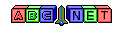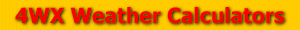# Wind Conversion

From the user, we are given a wind value and the unit to convert.

To convert between miles per hour (mph) and knots (kts):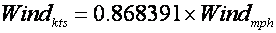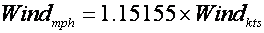To convert between miles per hour (mph) and meters per second (m/s):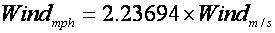To convert between miles per hour (mph) and feet per second (ft/s):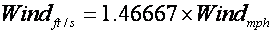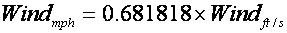To convert between miles per hours (mph) and kilometers per hour (km/h):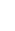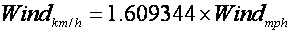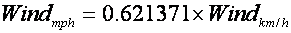To convert between knots (kts) and meters per second (m/s):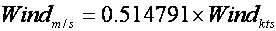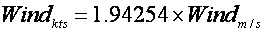To convert between knots (kts) and feet per second (ft/s):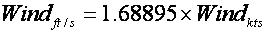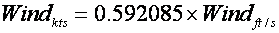To convert between knots (kts) and kilometers per hour (km/h):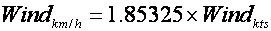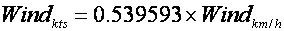To convert between meters per second (m/s) and feet per second (ft/s):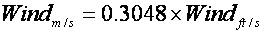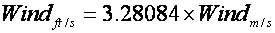To convert between meters per second (m/s) and kilometers per hour (km/h):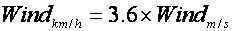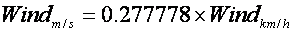To convert between feet per second (ft/s) and kilometers per hours (km/h):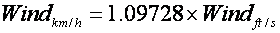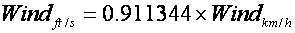Back to 4WX Weather Calculators index page

4WX Calculators Credit and disclaimer